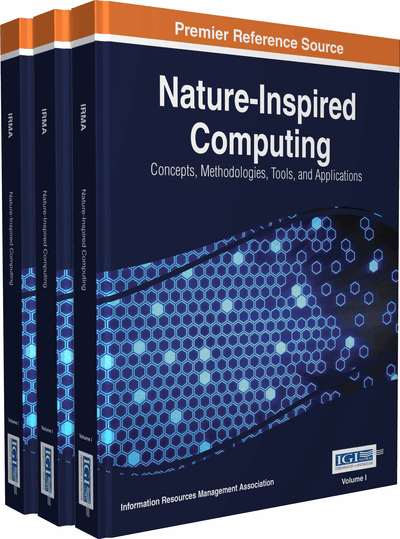# Artificial Sine and Cosine Trigonometric Higher Order Neural Networks for Financial Data Prediction

Ming Zhang (Christopher Newport University, USA)
DOI: 10.4018/978-1-5225-0788-8.ch028
Available
\$29.50
No Current Special Offers

## Abstract

This chapter develops two new nonlinear artificial higher order neural network models. They are Sine and Sine Higher Order Neural Networks (SIN-HONN) and Cosine and Cosine Higher Order Neural Networks (COS-HONN). Financial data prediction using SIN-HONN and COS-HONN models are tested. Results show that SIN-HONN and COS-HONN models are good models for financial data prediction compare with Polynomial Higher Order Neural Network (PHONN) and Trigonometric Higher Order Neural Network (THONN) models.
Chapter Preview
Top

## Introduction

The contributions of this chapter will be:

• Introduce the background of HONNs with the applications of HONNs in prediction area.

• Develop new HONN models called SIN-HONN and COS-HONN for financial data prediction.

• Provide the SIN-HONN and COS-HONN learning algorithm and weight update formulae.

• Compare SIN-HONN and COS-HONN with PHONN and THONN for data prediction.

This chapter is organized as follows: Section BACKGROUND gives the background knowledge of HONNs and introduction to applications using HONN in the data prediction area. Section SIN-HONN AND COS-HONN MODELS introduces both SIN-HONN structure and COS-HONN structure. Section LEARNING ALGORITHM OF SIN-HONN and COS-HONN MODELS provides the SIN-HONN model and COS-HONN model update formula, learning algorithms, and convergence theories. Section FINANCIAL DATA PREDICTION USING HONN MODELS compares SIN-HONN and COS-HONN models with other HONN models, and shows the results for data prediction using SIN-HONN and COS-HONN models.

Top

## Background

Artificial Neural Network (ANN) has been widely used in prediction area. Durbin and Rumelhart (1989) study a computationally powerful and biologically plausible extension to backpropagation networks. Yapo, Embrechets, and Cathey (1992) analyze prediction of critical heat using a hybrid kohon-backpropagation neural network intelligent. Moon and Chang (1994) experiment classification and prediction of the critical heat flux using fuzzy clustering and artificial neural networks. Charitou and Charalambous (1996) show the prediction of earnings using financial statement information with logic models and artificial neural networks. Doulamis, Doulamis, and Kollias (2000) research recursive nonlinear models for online traffic prediction of VBR MPEG Coded video sources. Atiya (2001) seeks bankruptcy prediction for credit risk using neural networks. Su, Fukuda, Jia, and Morita (2002) look into application of an artificial neural network in reactor thermo hydraulic problem which related to prediction of critical heat flux. Doulamis, Doulamis, and Kollias, (2003) revise an adaptable neural network model for recursive nonlinear traffic prediction and modeling of MPEG video sources. Sanchez, Alanis, and Rico (2004) expose electric load demand prediction using neural networks trained by Kalman filtering. Shawver (2005) scrutinize merger premium predictions using neural network approach.

Vaziri, Hojabri, Erfani, Monsey, and Nilforooshan (2007) disclose critical heat flux prediction by using radial basis function and multilayer perceptron neural networks. Welch, Ruffing, and Venayagamoorthy (2009) display comparison of feed-forward and feedback neural network architectures for short term wind speed prediction.

Artificial Higher Order Neural Network (HONN) has been widly used in the prediction areas as well. Saad, Prokhorov, and Wunsch (1998) provide comparative study of stock trend prediction using time delay recurrent and probabilistic neural networks. Zhang and Fulcher (2004) grant higher order neural networks for satellite weather prediction. Fulcher, Zhang, and Xu (2006) demonstrate an application of higher-order neural networks to financial time series prediction.

## Complete Chapter List

Search this Book:
Reset